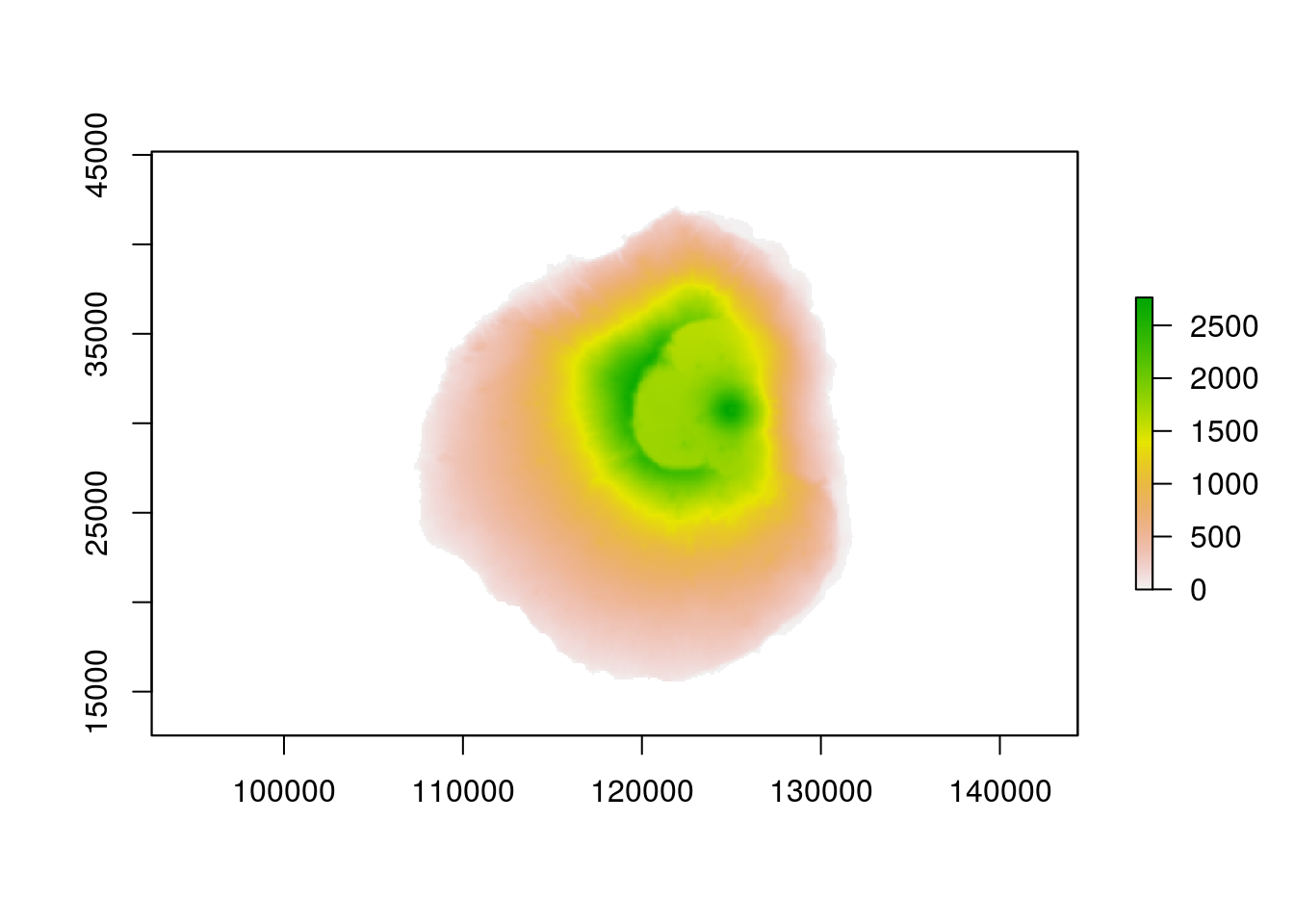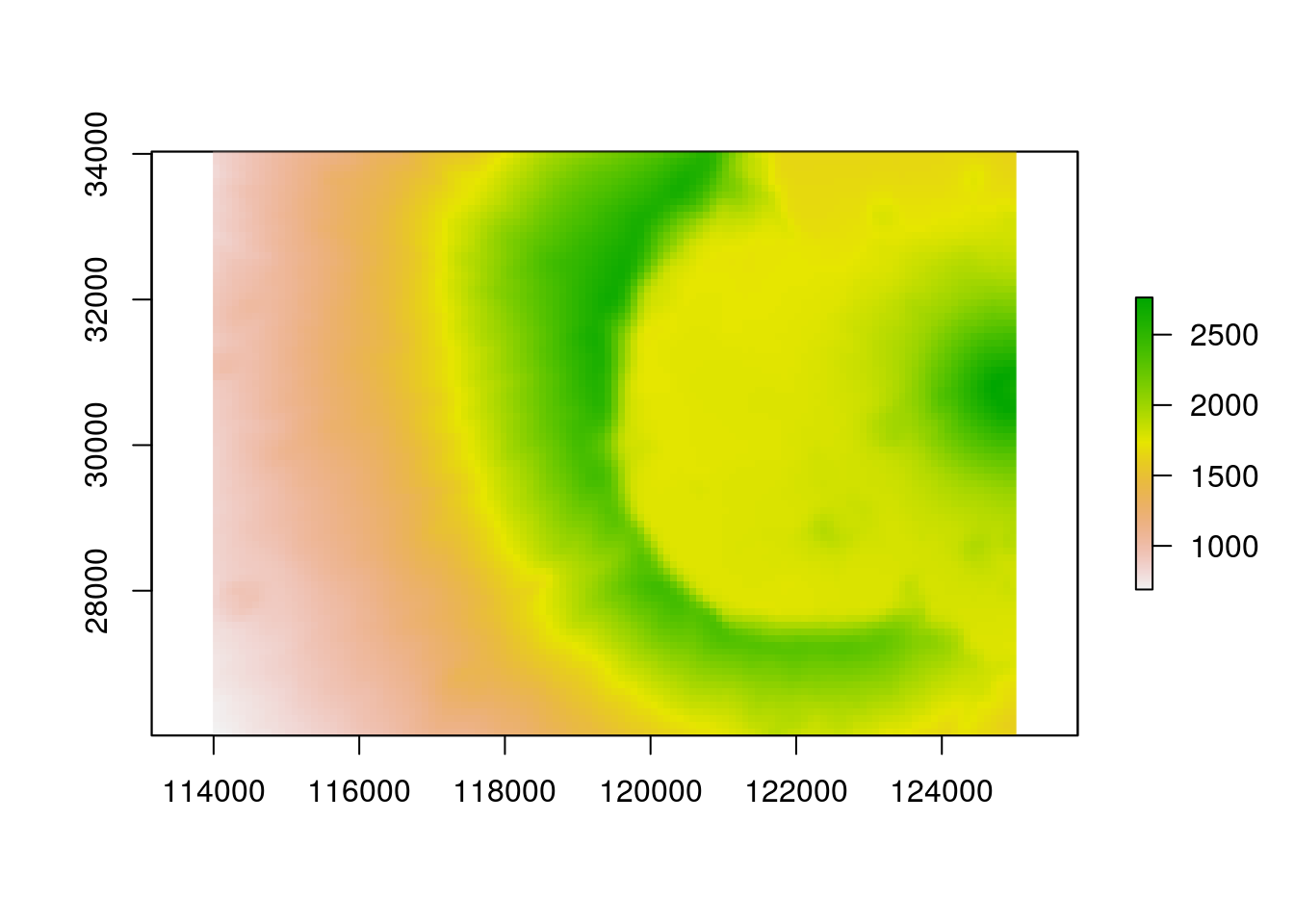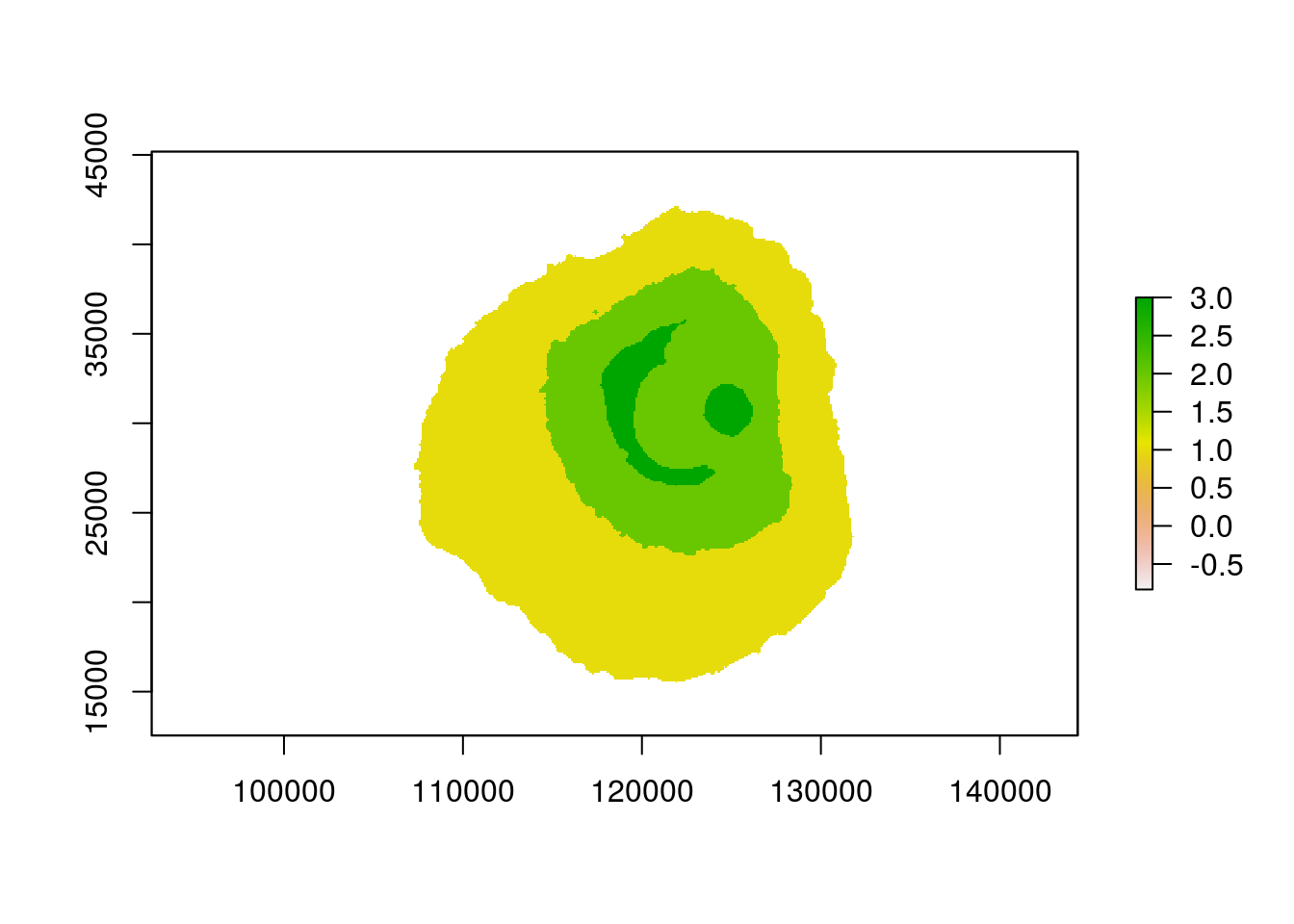#BIS-Fogo

### Site Tools

en:learning:schools:s02:code-examples:sm-ce-05-02

## C03-2: Working with raster images

#### Introduction

This tutorial gives some basic ideas about how to work with Raster data in R. As example, a digital evelation model from Fogo is used. In general, most functions which are available in ArcGIS, QGIS and so on are also available in R.

The most important package to work with rasters in R is the raster package. To handle projections, rgdal is necessary as well.

``library(raster)``
``## Loading required package: sp``
``library(rgdal)``
``````## rgdal: version: 1.0-4, (SVN revision 548)
##  Geospatial Data Abstraction Library extensions to R successfully loaded
##  Loaded GDAL runtime: GDAL 1.11.2, released 2015/02/10
##  Path to GDAL shared files: /usr/share/gdal/1.11
##  Loaded PROJ.4 runtime: Rel. 4.8.0, 6 March 2012, [PJ_VERSION: 480]
##  Path to PROJ.4 shared files: (autodetected)
##  Linking to sp version: 1.1-1``````

The easiest way to import a raster into R is to load a file which is already stored in a common raster format (e.g. tiff) on your computer

``dem <- raster("dem_fogo.tif")``

### Explore the data

The raster can be visualized using the normal plot function. The example in this tutorial is a digital elevation model of Fogo.

``plot(dem)``Use the following command to get all necessary information about your raster.

``print(dem)``
``````## class       : RasterLayer
## dimensions  : 354, 360, 127440  (nrow, ncol, ncell)
## resolution  : 89.7, 92.2  (x, y)
## extent      : 102329.4, 134621.4, 12550.29, 45189.09  (xmin, xmax, ymin, ymax)
## coord. ref. : +proj=lcc +lat_1=15 +lat_2=16.66666666666667 +lat_0=15.83333333333333 +lon_0=-24 +x_0=161587.83 +y_0=128511.202 +datum=WGS84 +units=m +no_defs +ellps=WGS84 +towgs84=0,0,0
## data source : /home/hanna/Documents/Lehre/kapVerde/dem_fogo.tif
## names       : dem_fogo
## values      : -0.8345093, 2763.871  (min, max)``````

### Crop a Raster

A raster can be cropped in different ways. You can either specify coordinates, or use a template to crop the raster, or do it interactively.

``dem_cropped <- crop(dem, drawExtent()) #the interactive way``

To do it in a reproduceable way, use coordinates instead of the interactive way

``````dem_cropped <- crop(dem, c(114000,125000,26000,34000)) #use coordinates
plot(dem_cropped)``````### Calculations with Raster data

You can do calculations with your raster in the same way as you use a calculator E.g.

``dem*2``

This command multiplied each value of dem by 2.

### Reclassifications

A reclassification of the values can be done using the reclassify function. However first, a reclasstable needs to be created. The reclass table in the following example can be interpreted in the way that all values from 0 to 1000 will become 1, all values from 1000 to 2000 will become 2 and all values between 2000 and 3000 will become 3. The result is a raster with only 3 classes. In this case, Fogo is classified according to its altitude.

``````rcl<- c(0,1000,1, 1000,2000,2,2000,3000,3)
dem_reclass <- reclassify(dem, rcl)``````### Calculate terrain characteristics

The raster package in R has several functions for terrain analysis. the most common functions calculate the aspect, the slope and the water flow direction using a digital elevation model as input. see ?terrain on how to interprete the flow direction.

``````terr_characteristics <- terrain(dem, opt=c("slope","aspect","flowdir"),unit='degrees')
print(terr_characteristics)``````
``````## class       : RasterBrick
## dimensions  : 354, 360, 127440, 3  (nrow, ncol, ncell, nlayers)
## resolution  : 89.7, 92.2  (x, y)
## extent      : 102329.4, 134621.4, 12550.29, 45189.09  (xmin, xmax, ymin, ymax)
## coord. ref. : +proj=lcc +lat_1=15 +lat_2=16.66666666666667 +lat_0=15.83333333333333 +lon_0=-24 +x_0=161587.83 +y_0=128511.202 +datum=WGS84 +units=m +no_defs +ellps=WGS84 +towgs84=0,0,0
## data source : in memory
## names       :       slope,      aspect,     flowdir
## min values  : 0.064284637, 0.003285333, 1.000000000
## max values  :    68.47605,   359.98697,   128.00000``````
``plot(terr_characteristics)``